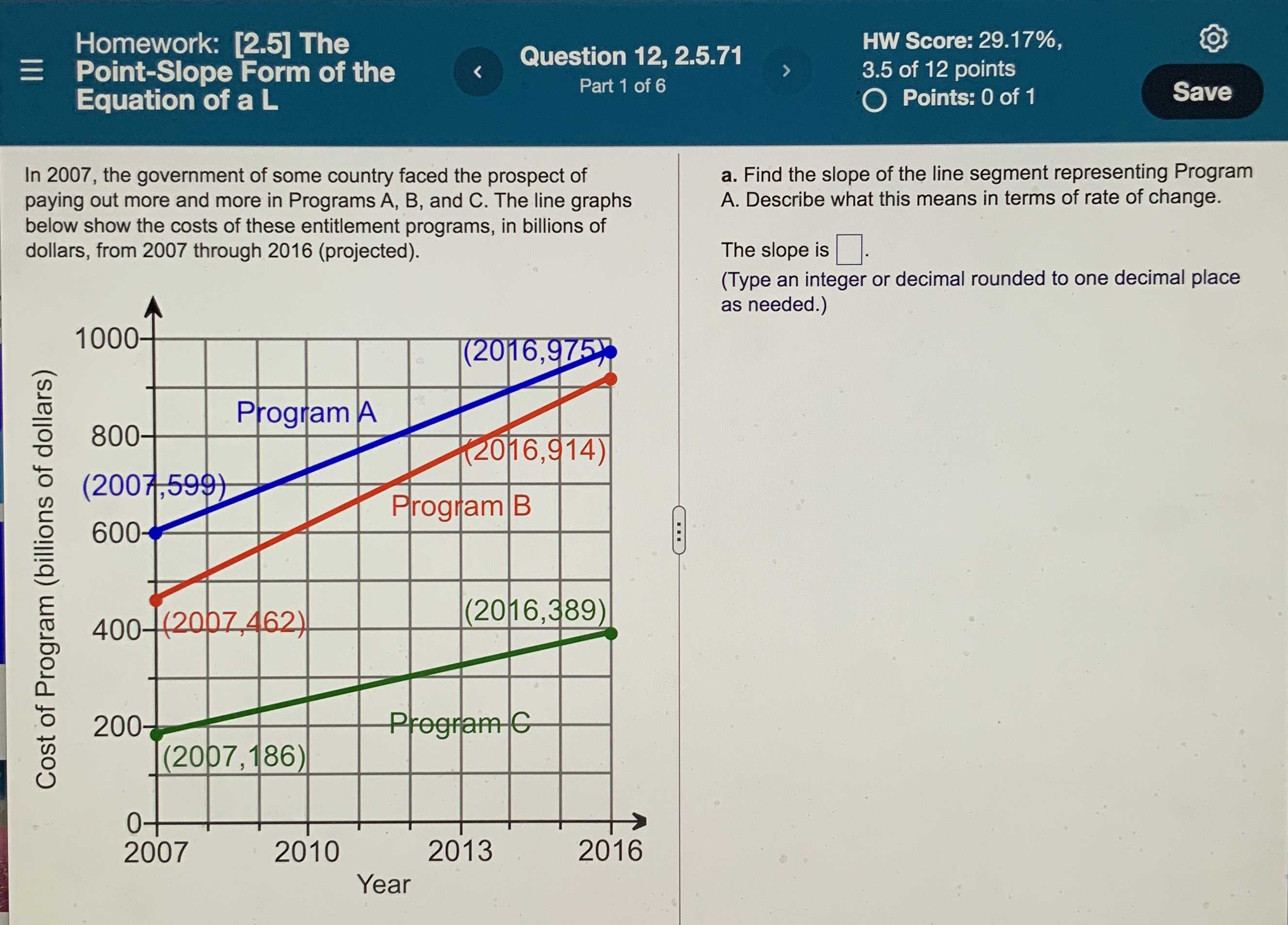### Still have math questions?

Algebra
QuestionIn $$2007$$ , the government of some country faced the prospect of paying out more and more in Programs A, B, and C. The line graphs below show the costs of these entitlement programs, in billions of dollars, from $$2007$$ through $$2016$$ (projected).

a. Find the slope of the line segment representing Program A. Describe what this means in terms of rate of change. The slope is $$\square$$ .

(Type an integer or decimal rounded to one decimal place as needed.)